### Restricting the Step Length

Almost all line-search algorithms use iterative extrapolation techniques which can easily lead them to (feasible) points where the objective function
is no longer defined. (e.g., resulting in indefinite matrices for ML estimation) or difficult to compute (e.g., resulting in floating point overflows). Therefore, PROC NLP provides options restricting the step lengthor trust region radius
, especially during the first main iterations.

The inner product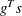of the gradientand the search directionis the slope of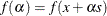along the search direction. The default starting value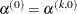in each line-search algorithm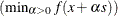during the main iterationis computed in three steps:

1. The first step uses either the difference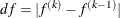of the function values during the last two consecutive iterations or the final step length value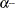of the last iteration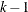to compute a first value of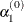.

• Not using the DAMPSTEP=option: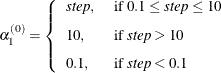with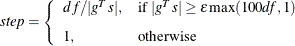This value ofcan be too large and lead to a difficult or impossible function evaluation, especially for highly nonlinear functions such as the EXP function.

• Using the DAMPSTEP=option: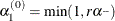The initial value for the new step length can be no larger thantimes the final step lengthof the previous iteration. The default value is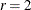.

2. During the first five iterations, the second step enables you to reduceto a smaller starting value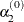using the INSTEP=option: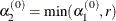After more than five iterations,is set to.

3. The third step can further reduce the step length by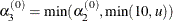whereis the maximum length of a step inside the feasible region.

The INSTEP=option lets you specify a smaller or larger radiusof the trust region used in the first iteration of the trust region, double dogleg, and Levenberg-Marquardt algorithms. The default initial trust region radius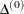is the length of the scaled gradient (Moré 1978). This step corresponds to the default radius factor of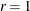. In most practical applications of the TRUREG, DBLDOG, and LEVMAR algorithms, this choice is successful. However, for bad initial values and highly nonlinear objective functions (such as the EXP function), the default start radius can result in arithmetic overflows. If this happens, you may try decreasing values of INSTEP=,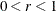, until the iteration starts successfully. A small factoralso affects the trust region radius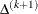of the next steps because the radius is changed in each iteration by a factor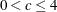, depending on the ratio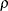expressing the goodness of quadratic function approximation. Reducing the radiuscorresponds to increasing the ridge parameter, producing smaller steps directed more closely toward the (negative) gradient direction.